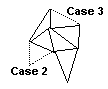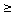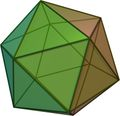# Regular Polyhedra

There are indeed only five regular (convex) polyhedra. And the fact was known to the ancient Greeks. Another term for the regular (convex) polyhedra is Platonic bodies. The fact is very well known and there is a great variety of different proofs to choose from. I like the one based on the Euler's formula. It's beautiful in its simplicity and admits generalizations to higher dimensions and more complex surfaces. But first we'll need a few definitions.### Definition 1

A polygon is an ordered collection of points A1, A2, ..., An and segments A1A2, A2A3, ..., AnA1 joining the points. The points and the segments are known as vertices and sides of the polygon. Oftentimes we talk about n-gons to emphasize the number of vertices (and of sides).

### Definition 2

A polygon whose vertices all belong to a single plane is called planar. Since I plan only to discuss planar polygons the term will be consistently omitted.

### Remark 1

Every polygon separates the plane into its interior (finite) and exterior (infinite) regions. It's a variant of the Jordan Curve Theorem that asserts the same property for a more general class of curves. The theorem that appears to be intuitively clear, was first stated by Camille Jordan (1838-1922) in his Cours d'Analyse. However the proof had gaps to fill which takes a considerable effort. It is simple although non-trivial for the polygonal case.

### Remark 2

Polygons are often identified with their interior regions.

### Definition 3

A polygon is said to be equilateral if its sides are all equal. It's said to be equiangular if its angles are all equal.

### Remark 3

A polygon can be equilateral without being equiangular and can be equiangular without being equilateral.

### Definition 4

A polygon that is both equilateral and equiangular is called regular. A regular n-gon is often denoted as {n}.

### Definition 5

Polyhedron is a solid (3D) body whose surface consists of a number of polygonal faces subject to two conditions to exclude some "abnormal" cases.

• every side of every polygon belongs to just one other polygon (this precludes T-"intersections" and the like)
• the faces that share a vertex form a chain of polygons in which every pair of consecutive polygons share a side (this precludes the case of two pyramids touching at a vertex)

### Remark 4

The sides of polygonal faces are called edges. Therefore, a polyhedron comprises three kinds of geometric objects - vertices, edges and faces.

### Definition 6

A polyhedron is said to be regular if all its faces are equal regular polygons and the same number of faces meet at every vertex. A polyhedron formed by the {p} polygons with q meeting at every vertex is denoted {p, q}.

### Definition 7

A shape (polygon or polyhedron or anything more general) is convex if with every pair of points that belong to the shape, the shape contains the whole straight line segment connecting the two points.

### Definition 8

A shape is said to be simple if it has no holes. For a polygon it means that every closed curve in its interior can be continuously shrunk into a point while the deformation is being carried entirely inside the polygon. For a polyhedron it means about the same thing with surface curves shrunk into a point while staying on the surface. Simple polyhedra can be continuously deformed into a sphere and, in addition, each of its faces is simple. The cube is simple.

### Remark 5

Convexity implies simplicity but not vice versa.The Euler's Theorem, also known as the Euler's formula, deals with the relative number of faces, edges and vertices that a polyhedron (or polygon) may have. Let, for a given polyhedron, F, E, V denote the number of faces, edges and vertices, respectively. Then we have the following

### Theorem 1 (Euler)

For a simple polyhedron F - E + V = 2. (This is also called the Euler's Formula.)

### Remark

The Theorem is due to Descartes (1639). It was rediscovered by Euler who published its proof in 1751. The proof below is due to Cauchy (1811).

### Proof of Theorem 1

Remove one face of the polyhedron. By pulling the edges of the missing face away from each other, deform all the rest into a planar network of points and curves. With no loss of generality it's possible to assume that the deformed edges remained straight line segments. Regular faces cease to be regular polygons if of course they were regular to start with. However, the number of vertices, edges and faces remained the same as those of the given polyhedron (the removed face corresponds to the exterior of the network.)

I am going to apply repeatedly a series of additional transformations that would simplify the network without changing its Euler's number (also Euler's characteristic) F - E + V.

1. If there is a polygonal face with more than three sides, we draw a diagonal. This adds one edge and one face. Continue adding edges until all the faces are triangular.
2. Remove (one at a time) all the triangles with two edges shared by the exterior of the network. This removes a vertex, two edges and one face.
3. Remove a triangle with only one edge adjacent to the exterior. This decreases the number of edges and faces by one each and does not change the number of vertices.Carry out steps 2 and 3 repeatedly one after another until only one triangle is left. For a single triangle F = 2 (counting the exterior), E = 3, V = 3. Therefore F - E + V = 2. Which proves the theorem.

### Theorem 2

There are only five regular convex polyhedra.

### Proof of Theorem 2

Assume there exists a {p, q} regular convex polyhedron. Since every face has p edges there would be a total of pF edges in all except that every edge is shared by two faces. Therefore pF = 2E. On the other hand, q edges meet at every vertex. Since each edge connects two vertices, qV = 2E. Substituting this into the Euler's formula gives

2E/p + 2E/q - E = 2 or 1/p + 1/q = 1/2 + 1/E

First of all, p≥3 and q3 since a polygon must have at least three vertices and three sides. p and q can't simultaneously be both greater than 3 because then the left hand side will be at most 1/4 + 1/4 = 1/2 < 1/2 + 1/E. Therefore, either p = 3 or q = 3. If p = 3,

1/q - 1/6 = 1/E

So that q can only be 3, 4 or 5. Solving the equation for E yields E equal 6, 12, or 30, respectively. Similarly, if r = 3 q can only be 3, 4 or 5 with E equal 6, 12 and 30 respectively. Then it all comes to five possible pairs. All five actually represent realizable shapes.

 Symbol F E V Name See also {3, 3} 4 6 4 TetrahedronGeoGebra Tetrahedron {3, 4} 8 12 6 OctahedronGeoGebra Octahedron {4, 3} 6 12 8 CubeGeoGebra Cube {3, 5} 20 30 12 IcosahedronGeoGebra Icosahedron {5, 3} 12 30 20 DodecahedronGeoGebra Dodecahedron

### Note

A recent book about platonic solids offers ready paper templates and clear instructions for creating platonic solids.## Generalizations

• ### N-dimensional spaces.

2-dimensional polygons have 1-dimensional sides. A 3-dimensional polyhedron has sides which are themselves 2-dimensional. a 4-dimensional polyhedron has sides that are themselves 3-dimensional polyhedra, etc. Let S, F, E, V be 3-dimensional sides, faces (2d), edges and vertices of a 4-dimensional polyhedron. Then S - F + E - V = 0.

In general, if an n-dimensional polyhedron has N0 vertices, N1 edges, N2 faces, etc. :-), then

N0 - N1 + N2 - ... + (-1)nNn = 1 - (-1)n-1.

This is known as the Schlafli formula.

• ### Not simple polyhedra.

The number of holes in a polyhedra is called its genus. For a surface of genus p, F - E + V = 2 - 2p.

• ### Not rectilinear surfaces.

It actually follows from the proof of Theorem 1 that the Theorem applies to what might be (and is) called maps and to which I referred to as networks. A plane map consists of nodes (vertices), edges connecting the nodes and countries (faces) bound by the edges. What would be the appropriate Euler's formula?## Problems

1. For a given polyhedron, let Fi be the number of i-gon faces. Then 3F3 + 4F4 + ... = 2E.
2. For a given polyhedron, let Vi be the number of vertices incident to i edges (i-valent vertices). Then 3V3 + 4V4 + ... = 2E.
3. (2V3 + 2V4 + ...) - (F3 + 2F4 + 3F5 + ...) = 4
4. (2F3 + 2F4 + ...) - (V3 + 2V4 + 3V5 + ...) = 4## References

1. R. Courant and H. Robbins, What is Mathematics?, Oxford University Press, 1996
2. H.S.M. Coxeter, Regular Polytopes, Dover Publications, NY, 1973
3. H.S.M. Coxeter, Introduction to Geometry, John Wiley & Sons, NY, 1961
4. M. Henle, A Combinatorial Introduction to Topology, Dover Publications, NY, 1994.
5. D. Hilbert and S. Cohn-Vossen, Geometry and Imagination, Chelsea Publishing Co, NY 1990.
6. I. Lakatos, Proofs and Refutations, Cambridge University Press, 1976
7. D. Radin, The Platonic Solids Book, CreateSpace Publishing, 2008
8. D. S. Richeson, Euler's Gem, Princeton University Press, 2008
9. D. Wells, You are a Mathematician, John Wiley & Sons, 1995## On Internet

Between them the sites below have so many further references it seems futile to locate a site not listed on one of these pages.